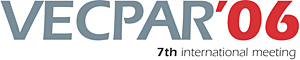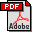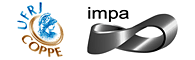vecpar.fe.up.pt/2006 | vecpar2006@fe.up.pt High Order Fourier-Spectral Solutions to Self Adjoint Elliptic Equations. Moshe Israeli (Technion-Israel Institute of Technology)Alexander Sherman (Technion-Israel Institute of Technology) Abstract: We develop a High Order Fourier solver for nonseparable, selfadjoint elliptic equations with variable (diffusion) coefficients. The solution of an auxiliary constant coefficient equation, serves in a transformation of the dependent variable. There results a "modified Helmholtz" elliptic equation with almost constant coefficients. The small deviations from constancy are treated as correction terms. We developed a highly accurate, fast, Fourier-spectral algorithm to solve such constant coefficient equations. A small number of correction steps is required in order to achieve very high accuracy. This is achieved by optimization of the coefficients in the auxiliary equation. For given coefficients the approximation error becomes smaller as the domain decreases. A highly parallelizable hierarchical procedure allows a decomposition into smaller sub-domains where the solution is efficiently computed. This step is followed by hierarchical matching to reconstruct the global solution. Numerical experiments illustrate the high accuracy of the approach even at coarse resolutions. Keywords: Numerical Methods (PDE), Parallel and Distributed Computing,Download the fullpaper TopRio de Janeiro | Brazil | 2006 | July | 10 11 12 13